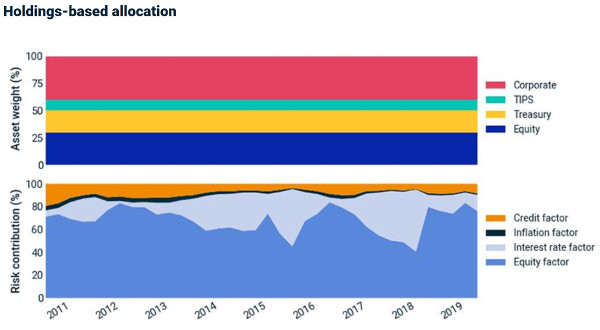﻿ A smoother ride? Looking at factor-based asset allocation | ETF Strategy - ETF Strategy

## A smoother ride? Looking at factor-based asset allocation

Sep 4th, 2019 | By | Category: Alternatives / Multi-Asset

By Andrea Amato, Associate, Fixed Income and Multi-Asset Class Research, and Chenlu Zhou, Vice President, Multi-Asset Class Factor Research Team at MSCI.A smoother ride? Looking at factor-based asset allocation

Strategic asset allocation has been an important driver of multi-asset-class portfolios’ returns. Traditionally, many investors have followed a holdings-based approach — where decisions are based on asset-class weights in the portfolio.

On the surface, this appears to produce a stable allocation, but is what you see always what you get?

In our example below, a factor-based approach resulted in less fluctuation in risk contributions and total portfolio risk than a holdings-based approach.

Stable asset-class weights ≠ stable risk contribution

We consider a hypothetical holdings-based allocation with a relatively low weight in equities compared to a 60/40 equity/fixed-income portfolio. Our hypothetical portfolio is designed to resemble the relatively low-equity asset allocation an insurance company might provide. The target weights are shown in the exhibit below.Source: MSCI.

We rebalance the hypothetical portfolio to the target weights on a quarterly basis, between Q1 2011 and Q1 2019. The exhibit below shows the stable-by-design asset weights (top) and the risk contributions (left) of equity, interest-rate, inflation and credit factors.

Two observations are notable:

First, with the equity allocation at just 30%, the risk contribution from equity averaged about 70% throughout the period.

Second, the factor risk contributions exhibited significant fluctuations. Most striking, by the beginning of 2018, a bull market pushed the equity risk contribution to a decade-low level of 40%, while the interest-rate factor risk contribution increased; however, rising risk in the aftermath of the February 2018 market sell-off quickly boosted the equity factor risk contribution to nearly 80%.

Steady asset weights, volatile risk levelsSource: MSCI.

What if, instead of setting asset-class weight targets, we set factor allocation targets, defined as the target risk contribution from each factor?

Since factor risk contributions add up to total portfolio risk, this effectively allowed the hypothetical investor in our example to assign a “risk budget” — rather than a “capital budget” — to each factor.

Intuitively, if investors expected that factor returns justified the amount of risk each factor contributes to the total portfolio risk, they could have inversely budgeted the risk contributions based on their expected factor returns.

Assuming relatively stable expected returns, a stable risk-contribution profile may have been desirable.

The exhibit below illustrates such a dynamic hypothetical portfolio composition (top), where asset-class weights responded to the changing factor regime and achieved a stable risk contribution (bottom).

Dynamic asset weights, steady risk levelsSource: MSCI.

We note that dynamic portfolio composition could result in a significantly higher portfolio turnover rate, which may be mitigated through constraints.

What about total risk and returns?

When it comes to risk and returns, the results were mixed. As we might expect, the factor-based allocation had more consistent total risk over the full period. However, as we see in the bottom panel of the exhibit below, which shows the Sharpe ratio (risk-adjusted return) on an annual rolling basis, each portfolio outperformed in different periods.

In 2013 and late 2017 the factor-based approach had higher risk-adjusted returns, while in 2015 and 2016 the holdings-based approach outperformed.

Further research can help us gain a more comprehensive understanding of the sources driving these behaviors, but intuitively one can understand when either approach performed better: The holdings-based approach was effectively a buy-losers, sell-winners strategy, which was advantageous when returns exhibited mean reversion — as the equity market did in 2016.

The factor-based approach, on the other hand, allocated more weight to an asset when the relevant factor volatility decreased. It outperformed while the low volatility lasted, as happened during late 2017’s persistent equity bull market.Source: MSCI.

Top panel shows the total risk of the two portfolios. The bottom panel shows the cumulative performance of the two portfolios (left axis), both scaled to an ex-ante volatility target of 4.7% at each rebalancing date.

By rescaling, we can compare the portfolio performance on a risk-adjusted basis. The bottom panel also presents the Sharpe ratio on an annual rolling basis (scatter plots, right axis).

Our analysis shows that the holdings-based asset-allocation approach used here resulted in greater-than-expected fluctuations in risk contributions. The factor-based approach, on the other hand, targeted a stable risk contribution by design, whereby asset-class weights varied in response to regime changes.

(The views expressed here are those of the author and do not necessarily reflect those of ETF Strategy.)